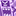# QlikView App Dev

Discussion Board for collaboration related to QlikView App Development.

Announcements
Join us for a live Q&A! September 21, 10 AM ET - Onboarding Fast in Qlik Sense SaaS! REGISTER
cancel
Showing results for
Did you mean:Not applicable

## Counting customers with certain variables in orders

Hi All,

I'm running into a problem. I want to count the number of customers who have ordered a certain set of clothes in their orders.

I at first made the following expression:

count({\$<%ARTICLE_VARIANT = {'A','B*','C','D'}, CUSTOMER =

p({\$<%ARTICLE_VARIANT = {'A'}>} CUSTOMER)*

p({\$<%ARTICLE_VARIANT = {'B*'}>} CUSTOMER)*

p({\$<%ARTICLE_VARIANT = {'C'}>} CUSTOMER)*

p({\$<%ARTICLE_VARIANT = {'D'}>} CUSTOMER)

>} DISTINCT CUSTOMER)

This gives me the number of customers who ordered all these articles. Now I have another set of different articles I would like to calculate as well. IE:

count({\$<%ARTICLE_VARIANT = {'D','E*','F','G'}, CUSTOMER =

p({\$<%ARTICLE_VARIANT = {'D'}>} CUSTOMER)*

p({\$<%ARTICLE_VARIANT = {'E*'}>} CUSTOMER)*

p({\$<%ARTICLE_VARIANT = {'F'}>} CUSTOMER)*

p({\$<%ARTICLE_VARIANT = {'G'}>} CUSTOMER)

>} DISTINCT CUSTOMER)

Now if I just sum these calculations I run into a problem. The result of the first can be 4 customers and of the second can be 4 customers, so the result would be 8. But what if a single customer ordered both sets. The result should be 7 instead of 8.  How can I do this?

1 Solution

Accepted SolutionsMVP

Maybe try to create a combined set the contains both customer sub sets, then do the DISTINCT count:

count(

{\$<%ARTICLE_VARIANT = {'A','B*','C','D'}, CUSTOMER =

p({\$<%ARTICLE_VARIANT = {'A'}>} CUSTOMER)*

p({\$<%ARTICLE_VARIANT = {'B*'}>} CUSTOMER)*

p({\$<%ARTICLE_VARIANT = {'C'}>} CUSTOMER)*

p({\$<%ARTICLE_VARIANT = {'D'}>} CUSTOMER)

>

+

<%ARTICLE_VARIANT = {'D','E*','F','G'}, CUSTOMER =

p({\$<%ARTICLE_VARIANT = {'D'}>} CUSTOMER)*

p({\$<%ARTICLE_VARIANT = {'E*'}>} CUSTOMER)*

p({\$<%ARTICLE_VARIANT = {'F'}>} CUSTOMER)*

p({\$<%ARTICLE_VARIANT = {'G'}>} CUSTOMER)

>}

DISTINCT CUSTOMER)

3 RepliesContributor III

Check out this description:

Combining logical operators in set analysisMVP

Maybe try to create a combined set the contains both customer sub sets, then do the DISTINCT count:

count(

{\$<%ARTICLE_VARIANT = {'A','B*','C','D'}, CUSTOMER =

p({\$<%ARTICLE_VARIANT = {'A'}>} CUSTOMER)*

p({\$<%ARTICLE_VARIANT = {'B*'}>} CUSTOMER)*

p({\$<%ARTICLE_VARIANT = {'C'}>} CUSTOMER)*

p({\$<%ARTICLE_VARIANT = {'D'}>} CUSTOMER)

>

+

<%ARTICLE_VARIANT = {'D','E*','F','G'}, CUSTOMER =

p({\$<%ARTICLE_VARIANT = {'D'}>} CUSTOMER)*

p({\$<%ARTICLE_VARIANT = {'E*'}>} CUSTOMER)*

p({\$<%ARTICLE_VARIANT = {'F'}>} CUSTOMER)*

p({\$<%ARTICLE_VARIANT = {'G'}>} CUSTOMER)

>}

DISTINCT CUSTOMER)Not applicable
Author

This worked! Thanks!Community Browser